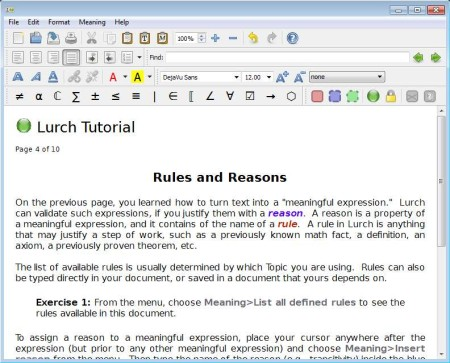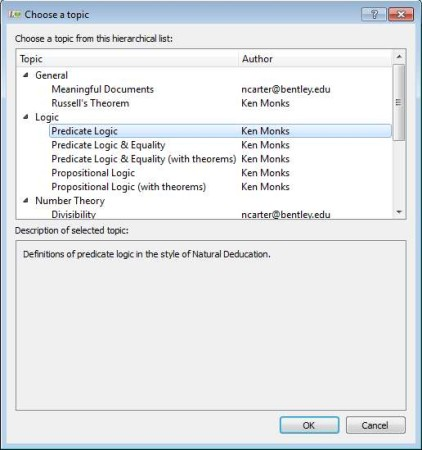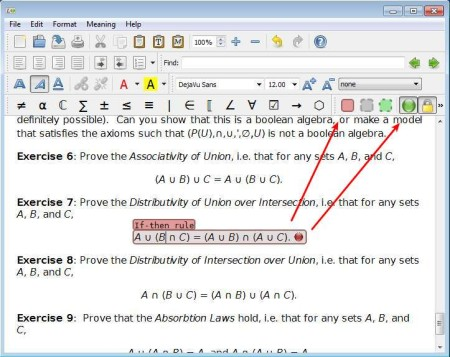Editor Ratings:
User Ratings:
[Total: 0 Average: 0]

Lurch is a free computer aided instruction software which will check how good you’ve written math problems in your text documents. This is actually a text editor, but instead of having a spell checker for checking grammar, Lurch will test mathematical equations, proofs, algebra, arithmetic and all the other expressions that are important for solving math problems.Interface of this free text editor with built-in math checker can be seen above. In appearance it’s not that much different from any other text editor. Toolbar at the top holds all the standard text formatting options. Differences from standard text editors are noticed when you move down, just above the document you can see a large selection of math symbols, which can be used when writing math proofs and equations. Key features of this free computer aided instructional text editor with built-in text editor are:

• Text editor – full set of text formatting tools, insert images, etc
• Mathematical expression checker for both logic and algebra problems
• Indicator which can mark on the fly errors in math – as you type
• Built-in rules for logic, number theory, set theory, and a few others
• Cross platform – works on Windows, Linux, BSD and Mac OS
• Simple and easy to use interface – large selection of math symbols

Lurch’s main goal is to allow instructors to create interactive math teaching aids. Lessons can be setup with the help of topics and also rules which are created within them. Rules are from where this free mathematical text editor will check if the math that the student writes is correct. In order for Lurch to work, you need to have an understanding of how the match expressions have to be written, and of course some understanding of math. Here’s a few pointers to help you get started.

Similar software: Lyx.

## How to write math problems and check them with Lurch

When you open up Lurch, you’ll be greeted with a short 10 step tutorial to help you get the hang of things. To write mathematical equations and expressions you can use the toolbar at the top. Something that’s more important would be topics, rules and reasons.There are some built-in topics, which contain rules that are gonna be used by the math checker. Setting a different topic means that different rules are gonna be applied, for example logic or number theory rules in the math problems that you’re writing. Set topic in File >> Choose topic.Before you can start checking if the math proofs and expressions that you’ve written are correct, you need to select them and then click on the green icon in the top right corner. This will tell Lurch that this isn’t just text and that it’s actually a math expression. Green icon where the arrow points is where you’ll turn on the checker. If there are problems, you should see a red indicator next to the expression that you’ve selected.

## Conclusion

There’s a lot more that you need to know to use Lurch. What we mentioned above is just the tip of the iceberg. If you’re teaching math and if you’re looking for something that might spark the interest of your students, this is just the things. Try it, free download.